# Skinny Saturday!

May 25 is the 4th Saturday of May, so that means it's Skinny Saturday!
Finish a bolt of regular priced fabric and take 25% off the price!

 table div table+table+table div table{width:100%;padding:0}table div table+table+table div table img{width:96.23%;padding:0;float:none}table div table+table+table div table td{width:100%;padding:0 1.88% 18px}/* styles */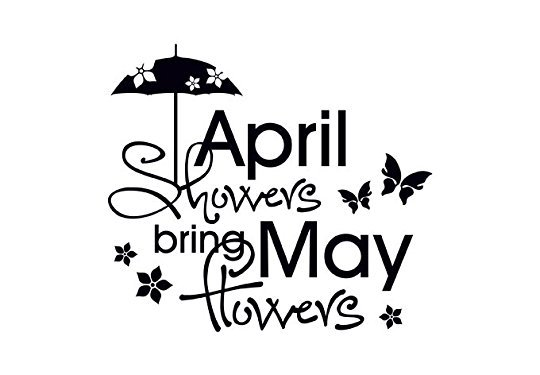# 9 Days Left in May!

If you have a blue envelope from shopping in April, bring it in by May 31 to see what your discount/prize is.

 table div table+table+table+table+table div table{width:100%;padding:0}table div table+table+table+table+table div table img{width:96.23%;padding:0;float:none}table div table+table+table+table+table div table td{width:100%;padding:0 1.88% 18px}/* styles */# Row by Row starts June 21!

Taste the Experience is the theme for 2019 and we have a very fun row planned!
Travel, collect and shop from June 21 to September 3.

 table div table+table+table+table+table+table+table div table{width:100%;padding:0}table div table+table+table+table+table+table+table div table img{width:96.23%;padding:0;float:none}table div table+table+table+table+table+table+table div table td{width:100%;padding:0 1.88% 18px}/* styles */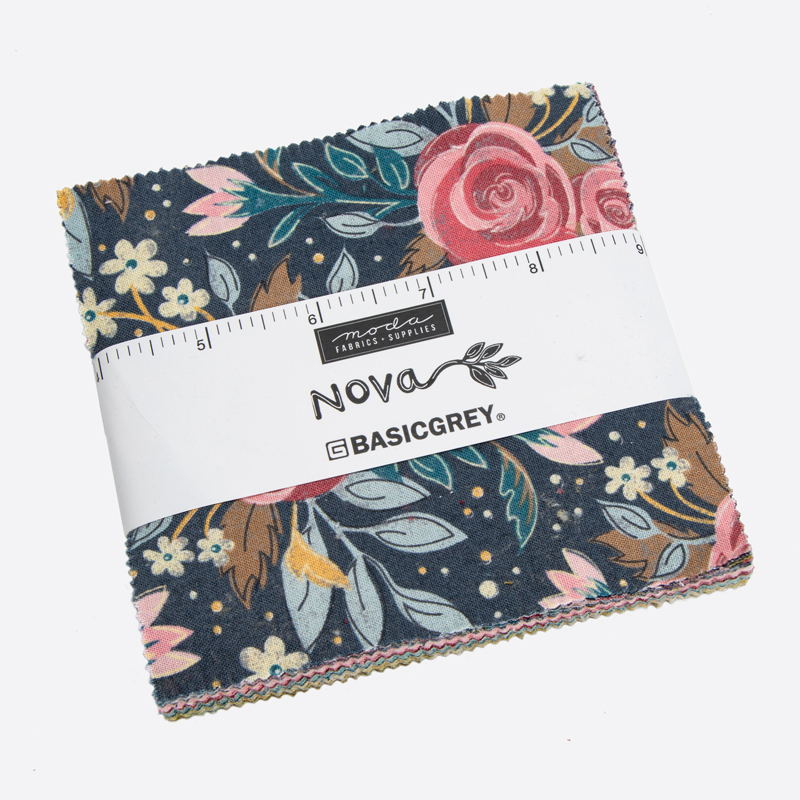# New Charm Packs!

Holiday, floral or Zen Chic, we have a great assortment of charm packs for you!

 table div table+table+table+table+table+table+table+table+table+table div table{width:100%;padding:0}table div table+table+table+table+table+table+table+table+table+table div table img{width:96.23%;padding:0;float:none}table div table+table+table+table+table+table+table+table+table+table div table td{width:100%;padding:0 1.88% 18px}/* styles */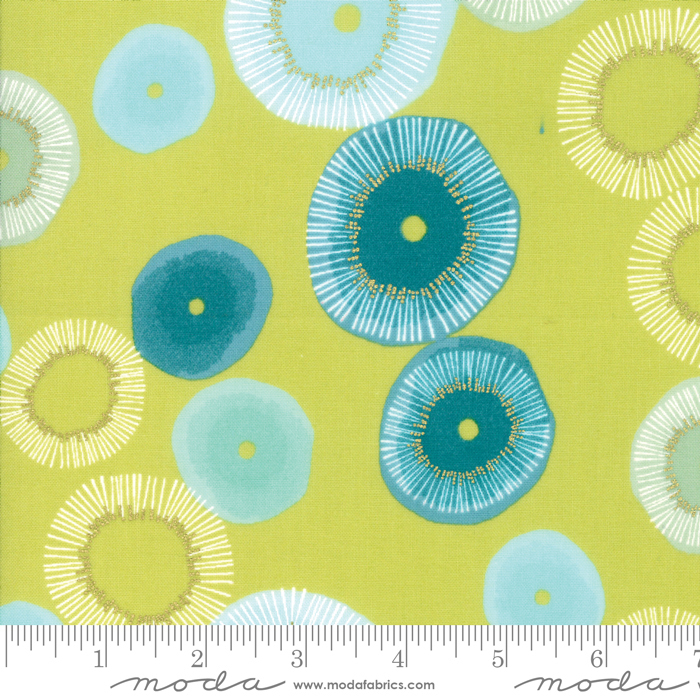# Day in Paris

The fabric is as lovely as the name!
Our favorite aquas and greens, with pinks and greys, this fabric is going fast!

 table div table+table+table+table+table+table+table+table+table+table+table+table+table div table{width:100%;padding:0}table div table+table+table+table+table+table+table+table+table+table+table+table+table div table img{width:96.23%;padding:0;float:none}table div table+table+table+table+table+table+table+table+table+table+table+table+table div table td{width:100%;padding:0 1.88% 18px}/* styles */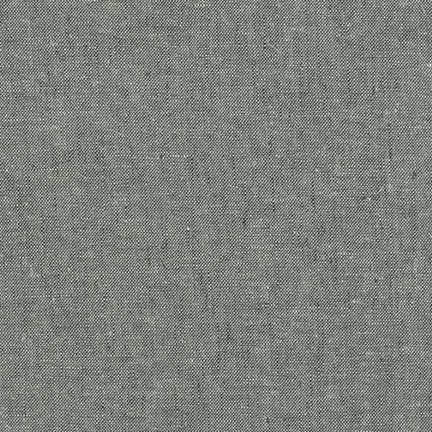# Essex Linen Restock!

Essex Linen by Robert Kaufman is a favorite around here.

Great background for table runners or quilts, it adds a texture without stealing the show.
Love it for Plenty of Pincushions too!

 table div table+table+table+table+table+table+table+table+table+table+table+table+table+table+table+table div table{width:100%;padding:0}table div table+table+table+table+table+table+table+table+table+table+table+table+table+table+table+table div table img{width:96.23%;padding:0;float:none}table div table+table+table+table+table+table+table+table+table+table+table+table+table+table+table+table div table td{width:100%;padding:0 1.88% 18px}/* styles */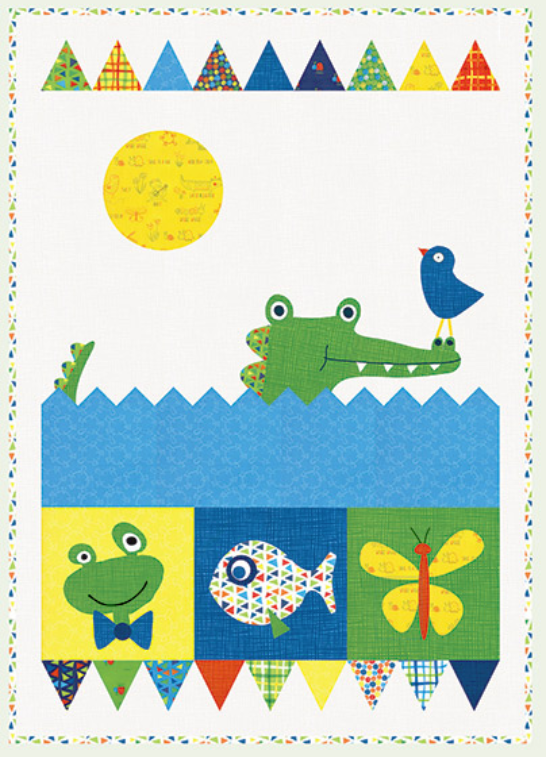# Lots of new goodies this week!

Our Later Alligator kit are ready. This adorable quilt measures 36″ x 52″ finished size – perfect for kids! The waves, alligator scutes, and teeth are dimensional for added fun!

New issue of Quiltmania and Quilt Sampler!

Down by the Sea Embroidery Designs

New Kimberbell Bench Buddies
and more!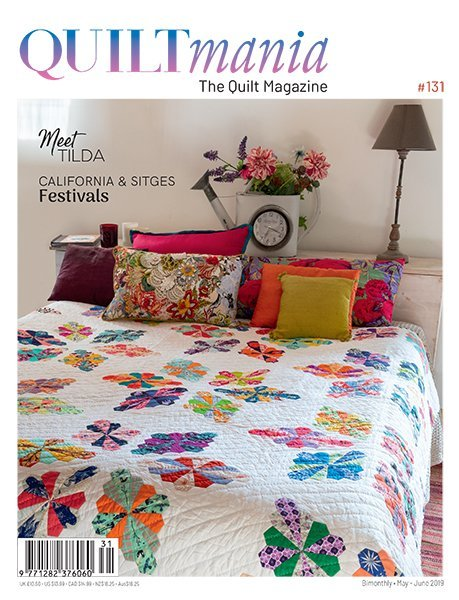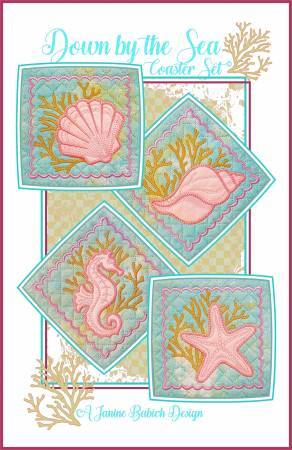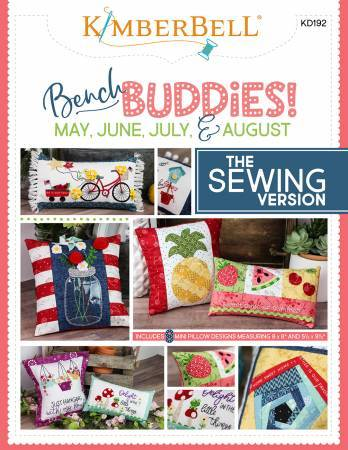table div table+table+table+table+table+table+table+table+table+table+table+table+table+table+table+table+table+table+table+table div table{width:100%;padding:0}table div table+table+table+table+table+table+table+table+table+table+table+table+table+table+table+table+table+table+table+table div table img{width:96.23%;padding:0;float:none}table div table+table+table+table+table+table+table+table+table+table+table+table+table+table+table+table+table+table+table+table div table td{width:100%;padding:0 1.88% 18px}/* styles */Soiree means "evening party"

# Sunday Soiree!

Did you catch our FB Live last Sunday? It was so much fun, we are going to do it every Sunday night!

# It's a party on Facebook and you are invited!

Sunday night get comfy, get your beverage of choice and get excited about all the fun things I am going to show you on Facebook Live!
You will be able to purchase during the Live Broadcast and we can ship - sort of like Stuff Your Stocking but all in one night.
I'm going to show you fabrics, notions, kits and other great things that you need to know about!
There will be live demos and first-look at new products!
We will go "live" at 6:55 and see what the hounds are up to, then
start the Soiree at 7pm (Eastern)

## Join me for a fun evening!

 table div table+table+table+table+table+table+table+table+table+table+table+table+table+table+table+table+table+table+table+table+table+table+table+table+table div table{width:100%;padding:0}table div table+table+table+table+table+table+table+table+table+table+table+table+table+table+table+table+table+table+table+table+table+table+table+table+table div table img{width:96.23%;padding:0;float:none}table div table+table+table+table+table+table+table+table+table+table+table+table+table+table+table+table+table+table+table+table+table+table+table+table+table div table td{width:100%;padding:0 1.88% 18px}/* styles */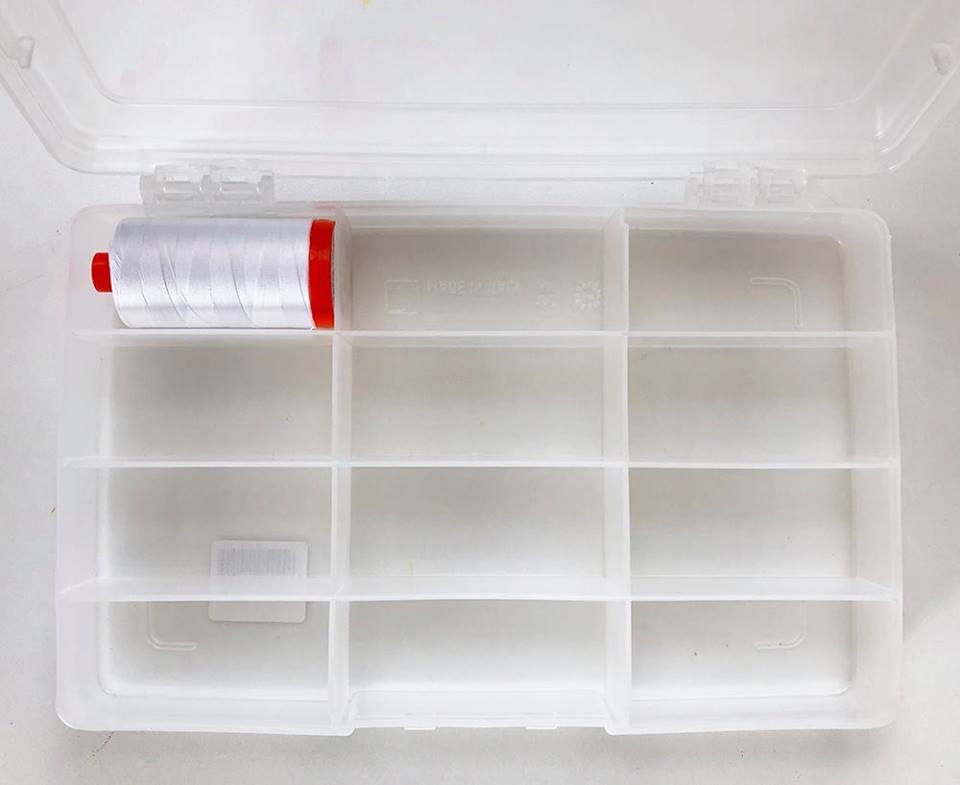# Aurifil Thread Club!

## Best deal!

Purchase a thread case, containing one spool of white Aurifil thread #2024 for \$15, every time you bring your thread case back to the shop, you get 15% off Aurifil threads!
Fabulous deal on the best threads!
(Instore only)

 table div table+table+table+table+table+table+table+table+table+table+table+table+table+table+table+table+table+table+table+table+table+table+table+table+table+table+table div table{width:100%;padding:0}table div table+table+table+table+table+table+table+table+table+table+table+table+table+table+table+table+table+table+table+table+table+table+table+table+table+table+table div table img{width:96.23%;padding:0;float:none}table div table+table+table+table+table+table+table+table+table+table+table+table+table+table+table+table+table+table+table+table+table+table+table+table+table+table+table div table td{width:100%;padding:0 1.88% 18px}/* styles */## In Need of some Stitch Therapy?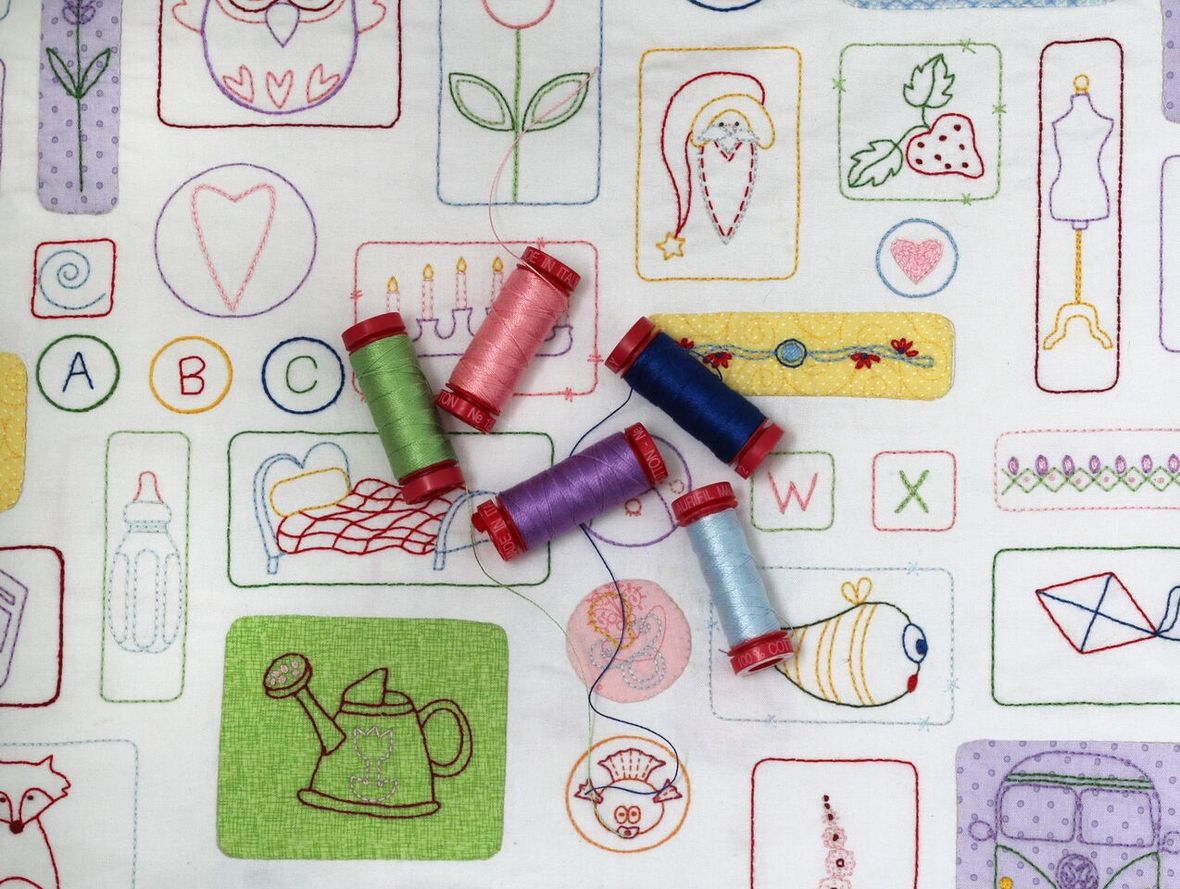# ONLY 1 panel left!

It's not too late!
We have 1 panel and 1 thread kit AND...
your 365 days start when you get signed up, so no playing catch up, just a little stitching every day.

 table div table+table+table+table+table+table+table+table+table+table+table+table+table+table+table+table+table+table+table+table+table+table+table+table+table+table+table+table+table+table div table{width:100%;padding:0}table div table+table+table+table+table+table+table+table+table+table+table+table+table+table+table+table+table+table+table+table+table+table+table+table+table+table+table+table+table+table div table img{width:96.23%;padding:0;float:none}table div table+table+table+table+table+table+table+table+table+table+table+table+table+table+table+table+table+table+table+table+table+table+table+table+table+table+table+table+table+table div table td{width:100%;padding:0 1.88% 18px}/* styles */# Next PMS Night is Friday, June 14

6pm to midnight
Treat yourself to a night of food, fabric and friends!
Who knows, you could be the next fat quarter winner!

 table div table+table+table+table+table+table+table+table+table+table+table+table+table+table+table+table+table+table+table+table+table+table+table+table+table+table+table+table+table+table+table+table+table div table{width:100%;padding:0}table div table+table+table+table+table+table+table+table+table+table+table+table+table+table+table+table+table+table+table+table+table+table+table+table+table+table+table+table+table+table+table+table+table div table img{width:96.23%;padding:0;float:none}table div table+table+table+table+table+table+table+table+table+table+table+table+table+table+table+table+table+table+table+table+table+table+table+table+table+table+table+table+table+table+table+table+table div table td{width:100%;padding:0 1.88% 18px}/* styles */# Casual Sew 10am to 4pm

Friday, May 24, 31, June 7, 14, 21, 28

# Upcoming Classes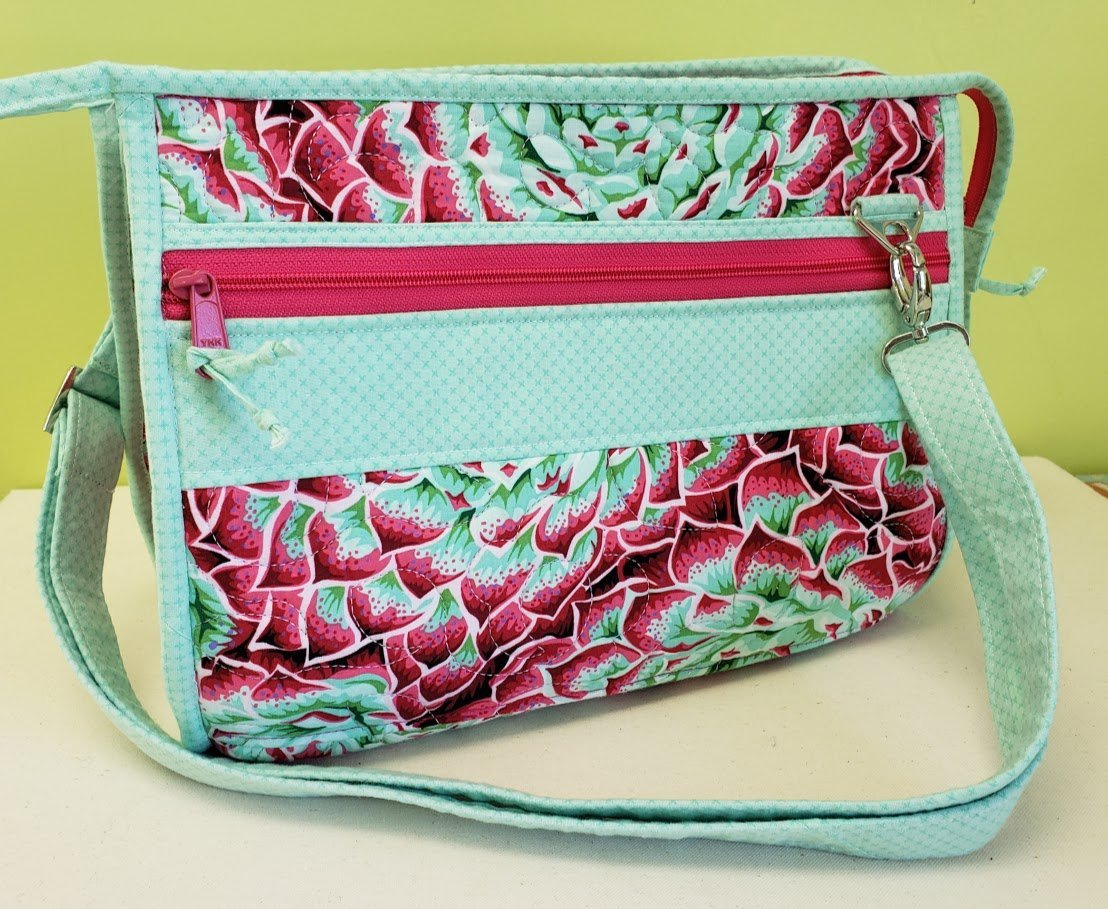# Take a Stand

Learn to make a spacious "stand up" tote!
Class is Saturday, June 1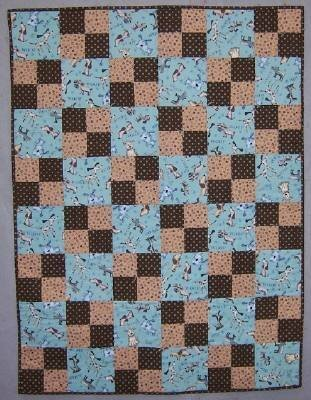# Beginning Quilt Making

If you have always wanted to make a quilt, this 5 session class is for you!
Class starts Tuesday, June 4

# And there's more!

 table div table+table+table+table+table+table+table+table+table+table+table+table+table+table+table+table+table+table+table+table+table+table+table+table+table+table+table+table+table+table+table+table+table+table+table+table+table+table+table+table+table+table div table{width:100%;padding:0}table div table+table+table+table+table+table+table+table+table+table+table+table+table+table+table+table+table+table+table+table+table+table+table+table+table+table+table+table+table+table+table+table+table+table+table+table+table+table+table+table+table+table div table img{width:96.23%;padding:0;float:none}table div table+table+table+table+table+table+table+table+table+table+table+table+table+table+table+table+table+table+table+table+table+table+table+table+table+table+table+table+table+table+table+table+table+table+table+table+table+table+table+table+table+table div table td{width:100%;padding:0 1.88% 18px}/* styles */# Open 7 days a week for your convenience!

Monday through Saturday, 10am to 5pm
Sunday 12pm to 4pm

See you soon!

## Remember to join us for the Sunday Soiree on Facebook!

 table div table+table+table+table+table+table+table+table+table+table+table+table+table+table+table+table+table+table+table+table+table+table+table+table+table+table+table+table+table+table+table+table+table+table+table+table+table+table+table+table+table+table+table+table div table{width:100%;padding:0}table div table+table+table+table+table+table+table+table+table+table+table+table+table+table+table+table+table+table+table+table+table+table+table+table+table+table+table+table+table+table+table+table+table+table+table+table+table+table+table+table+table+table+table+table div table img{width:96.23%;padding:0;float:none}table div table+table+table+table+table+table+table+table+table+table+table+table+table+table+table+table+table+table+table+table+table+table+table+table+table+table+table+table+table+table+table+table+table+table+table+table+table+table+table+table+table+table+table+table div table td{width:100%;padding:0 1.88% 18px}/* styles */